Math resources Geometry Volume

Volume of a cube

# Volume of a cube

Here you will learn about the volume of a cube, including how to calculate the volume of a cube within mathematical problems and within real-world contexts.

Students will first learn about volume of a cube as part of measurement and data in 5th grade and extend their learning as part of geometry in 6th grade.

## What is the volume of a cube?

The volume of a cube is the amount of space there is within a cube.

A cube is a three-dimensional shape with 6 square faces.

To find the volume of a cube, with side length a, you can use the volume of a cube formula, \text {Volume }=a^{3}.

Volume is measured in cubic units. For example, cubic inches (in^3), cubic meters (m^3), or cubic centimeters (cm^3).

For example,

The volume of this cube is,

volume = a^3

volume = 8^3

volume = 512 \, cm^3

The length, width, and height of the cube are multiplied together to find the total volume.

### What is the volume of a cube?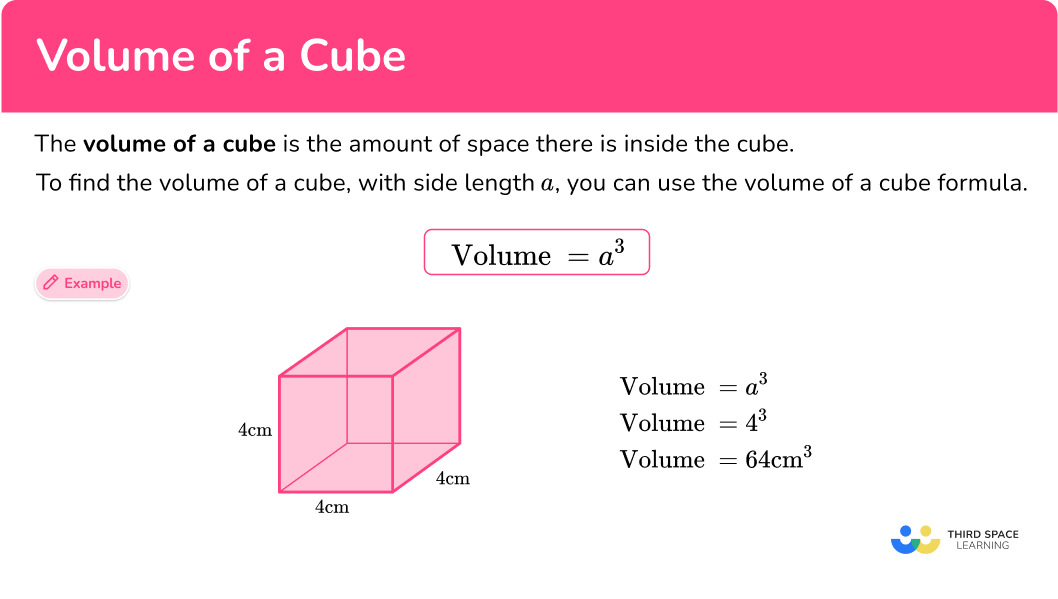## Common Core State Standards

How does this relate to 5th grade math and 6th grade math?

• Grade 5 – Measurement and Data (5.MD.3)
Recognize volume as an attribute of solid figures and understand concepts of volume measurement.

a. A cube with side length 1 unit, called a “unit cube,” is said to have “one cubic unit” of volume, and can be used to measure volume.

b. A solid figure which can be packed without gaps or overlaps using n unit cubes is said to have a volume of n cubic units.

• Grade 5 – Measurement and Data (5.MD.4)
Measure volumes by counting unit cubes, using cubic cm, cubic in, cubic ft, and improvised units.

• Grade 5 – Measurement and Data (5.MD.5)
Relate volume to the operations of multiplication and addition and solve real world and mathematical problems involving volume.

a. Find the volume of a right rectangular prism with whole-number side lengths by packing it with unit cubes, and show that the volume is the same as would be found by multiplying the edge lengths, equivalently by multiplying the height by the area of the base. Represent threefold whole-number products as volumes, for example, to represent the associative property of multiplication.

b. Apply the formulas V = l \times w \times h and V = b \times h for rectangular prisms to find volumes of right rectangular prisms with whole number edge lengths in the context of solving real world and mathematical problems.

c. Recognize volume as additive. Find volumes of solid figures composed of two non-overlapping right rectangular prisms by adding the volumes of the non-overlapping parts, applying this technique to solve real world problems.

• Grade 6 – Geometry (6.G.2)
Find the volume of a right rectangular prism with fractional edge lengths by packing it with unit cubes of the appropriate unit fraction edge lengths, and show that the volume is the same as would be found by multiplying the edge lengths of the prism.

Apply the formulas V = l \times w \times h and V = b \times h to find volumes of right rectangular prisms with fractional edge lengths in the context of solving real-world and mathematical problems.

## How to calculate the volume of a cube

In order to calculate the volume of a cube:

1. Write down the formula.
\text{Volume }=a^{3}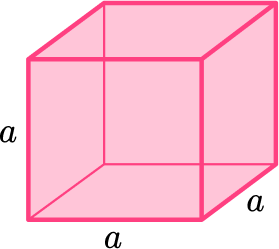2. Substitute the values into the formula.
3. Work out the calculation.
4. Write the answer and include the units.

## Volume of a cube examples

### Example 1: volume of a cube

Find the volume of the cube.

1. Write down the formula.

\text{Volume }=a^{3}

2Substitute the values into the formula.

Here, the sides of the cube are 6 \, cm.

\text{Volume }=6^{3}

3Work out the calculation.

\begin{aligned} \text{Volume} &=6 \times 6 \times 6\\\\ &=216 \end{aligned}

4Write the answer and include the units.

The measurements are in centimeters. Therefore, the volume will be in cubic centimeters.

\text{Volume }=216 \mathrm{~cm}^{3}

### Example 2: volume of a cube

Find the volume of this cube.

\text{Volume }=a^{3}

Here, the edges are each 7 \, in.

\text{Volume }=7^{3}

\begin{aligned} \text{Volume} &=7 \times 7 \times 7\\\\ &=343 \end{aligned}

The measurements are in inches. Therefore, the volume will be in cubic inches.

\text{Volume }=343 \mathrm{~in}^{3}

### Example 3: volume of a cube – different units

Find the volume of this cube.

\text{Volume }=a^{3}

Notice here that one of the units is in centimeters while the other is in meters. You need all the units to be the same to calculate the volume.

This is a cube, so you know all the edges are the same length, so you can easily change meters to centimeters.

0.6 \mathrm{~m}=60 \mathrm{~cm}

\text{Volume }=60^{3}

\begin{aligned} Volume &=60×60×60 \\\\ &=216,000 \end{aligned}

The measurements are in centimeters. Therefore, the volume will be in cubic centimeters.

\text{Volume }=216,000 \mathrm{~cm}^{3}

### Example 4: volume of a cube – different units

\text{Volume }=a^{3}

Notice here that one of the units is in meters, one is centimeters, and another is in millimeters. You need all the units to be the same to calculate the volume.

This is a cube, so you know all the edges are the same length, so you can easily change the centimeters and the millimeters to meters.

\begin{aligned} & 15,000 \mathrm{~mm}=15 \mathrm{~m} \\\\ & 1,500 \mathrm{~cm}=15 \mathrm{~m} \end{aligned}

\begin{aligned} \text { Volume } & =15 \times 15 \times 15 \\\\ & =3,375 \end{aligned}

The measurements are in meters. Therefore, the volume will be in cubic meters.

\text{ Volume }=3,375 \mathrm{~m}^3

### Example 5: volume of a cube – word problem

Anna has a Rubik’s cube. Each edge of the Rubik’s cube is 5.8 \, cm. What is the volume of the Rubik’s cube?

\text{Volume }=a^{3}

Here, the edges are each 5.8 \, cm.

\text{ Volume }=5.8^3

\begin{aligned} \text { Volume } & =5.8 \times 5.8 \times 5.8 \\\\ & =195.112 \end{aligned}

The measurements are in centimeters. Therefore, the volume will be in cubic centimeters.

\text { Volume }=195.112 \mathrm{~cm}^3

### Example 6: volume of a cube – word problem

Grant is moving to a new house and needs to buy boxes to pack his belongings into. He wants to know the volume of the cube-shaped box shown below. The length of the side of the box is 20 inches. Help him determine the box’s volume.

\text{Volume }=a^{3}

Here, the edges are each 20 \, in.

\text{Volume }=20^{3}

\begin{aligned} \text { Volume } & =20 \times 20 \times 20 \\\\ & =8,000 \end{aligned}

The measurements are in inches. Therefore, the volume will be in cubic inches.

\text{Volume }=8,000 \mathrm{~in}^3

### Teaching tips for volume of a cube

• Be sure to give students a wide variety of practice problems within their worksheets that incorporate cubes and other three-dimensional objects found in the real world. This will give students a deeper understanding of the concept of volume.

• Students should fully understand why the volume formula works with a cube – they need to understand why multiplying 3 of the cubes edges (length, width and height) gives us the cube’s volume. Once they have a strong understanding of the formula, they can easily use it to find the volume of any cube.

### Easy mistakes to make

• Forgetting to include the units in your answer or writing the incorrect units.
Volume is measured in cubic units. (For example, mm^3, cm^3, m^3, etc).

• Not converting all measurements to the same unit
You need to make sure all measurements are in the same units before calculating volume.
For example, you can’t have some measurements in centimeters and some in meters.

• Confusing volume of a cube with surface area of a cube
Volume is the space inside a cube and it is a three-dimensional measurement. Surface area is the total area of each of the cube’s square faces and it is a two-dimensional measurement.

### Practice volume of a cube questions

1. Find the volume of the cube.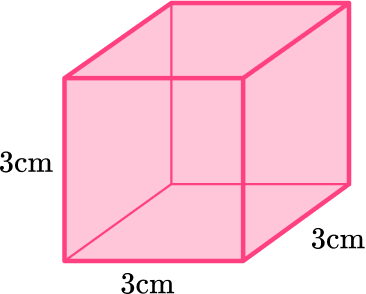9 \mathrm{~cm}^{3}27 \mathrm{~cm}^{3}54 \mathrm{~cm}^{3}36 \mathrm{~cm}^{3}\begin{aligned} \text{Volume } &=a^{3} \\\\ \text{Volume } &=3^{3} \\\\ &=3 \times 3 \times 3 \\\\ &=27 \mathrm{~cm}^{3} \end{aligned}

2. Find the volume of the cube.1.25 \mathrm{~m}^{3}150 \mathrm{~m}^{3}125 \mathrm{~m}^{3}0.125 \mathrm{~m}^{3}\begin{aligned} \text{Volume } &=a^{3} \\\\ \text{Volume }&=0.5^{3}\\\\ &=0.5 \times 0.5 \times 0.5\\\\ &=0.125 \mathrm{~m}^{3} \end{aligned}

3. Find the volume of this cube.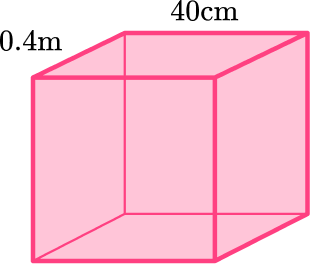64,000 \mathrm{~cm}^{3}96 \mathrm{~cm}^{3}64 \mathrm{~cm}^{3}6.4 \mathrm{~cm}^{3}Notice here that one of the units is in centimeters while the other is in meters. You need all the units to be the same to calculate the volume.

This is a cube, which means all the edges are the same length, so you can easily change meters to centimeters, 0.4m = 40 \, cm.

\begin{aligned} \text{Volume } &=a^{3} \\\\ \text{Volume }&=40^{3}\\\\ &=40 \times 40 \times 40\\\\ &=64,000 \end{aligned}

The measurements are in centimeters. Therefore, the volume will be in cubic centimeters.

\text{Volume }=64,000 \mathrm{~cm}^{3}

4. Kara has 5 sugar cubes to put into her coffee. Each sugar cube has side lengths of 13 \, mm. What is the total volume of all 5 sugar cubes?

2,197 \mathrm{~mm}^{3}65 \mathrm{~mm}^{3}10,985 \mathrm{~mm}^{3}195 \mathrm{~mm}^{3}First, you need to find the volume of one sugar cube.

\begin{aligned} \text{Volume } &=a^{3} \\\\ \text { Volume } & =13^3 \\\\ &= 13 \times 13 \times 13 \\\\ &= 2,197 \mathrm{~mm}^3 \end{aligned}

Since each sugar cube has the same side lengths, you can multiply the volume of one sugar cube by 5 to find the total volume of all 5 sugar cubes.

2,197 \mathrm{~mm}^3 \times 5=10,985 \mathrm{~mm}^3

5. Vera has two boxes, box A and box B, which are shown below. How much greater is the volume of box B than the volume of box A?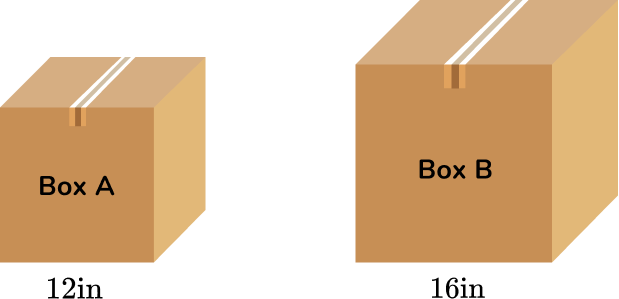1,728 \mathrm{~in}^{3}4,096 \mathrm{~in}^{3}5,824 \mathrm{~in}^{3}2,368 \mathrm{~in}^{3}First, you need to find the volume of box A and the volume of box B. Then you need to find the difference between the two.

Box A :

\begin{aligned} \text{Volume } &=a^{3} \\\\ \text { Volume } & =12^3 \\\\ & = 12 \times 12 \times 12 \\\\ & = 1,728 \mathrm{~in}^3 \end{aligned}

Box B :

\begin{aligned} \text{Volume } &=a^{3} \\\\ \text { Volume } & =16^3 \\\\ &= 16 \times 16 \times 16 \\\\ &= 4,096 \mathrm{~in}^3 \end{aligned}

4,096-1,728=2,368

The volume of box B is 2,368 \mathrm{~in}^3 greater than the volume of box A.

6. This sculpture is formed by placing one cube on top of another. Find the total volume of the sculpture.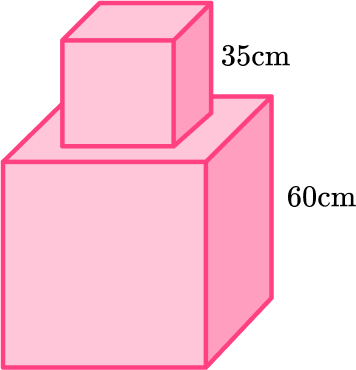216, 000 \mathrm{~cm}^{3}258,875 \mathrm{~cm}^{3}42, 875 \mathrm{~cm}^{3}143, 125 \mathrm{~cm}^{3}To find the total volume of the sculpture, you need to find the volume of the top cube and the volume of the bottom cube, then add the volumes together.

Volume of bottom cube: 60 \times 60 \times 60=216,000 \mathrm{~cm}^3

Volume of top cube: 35 \times 35 \times 35=42,875 \mathrm{~cm}^3

Total volume: 216,000+42,875=258,875 \mathrm{~cm}^3

## Volume of a cube FAQs

What is a cube?

A cube is a three-dimensional shape with 6 square faces. The edges of the cube are of equal length.

How do you find the volume of a cube?

To find the volume of a cube, with side length a, you can use the volume of a cube formula, \text { Volume }=a^3. This is the same as multiplying length times width times height.

What is the formula for the volume of a cube?

The volume of a cube formula is \text { Volume }=a^3.

## Still stuck?

At Third Space Learning, we specialize in helping teachers and school leaders to provide personalized math support for more of their students through high-quality, online one-on-one math tutoring delivered by subject experts.

Each week, our tutors support thousands of students who are at risk of not meeting their grade-level expectations, and help accelerate their progress and boost their confidence.